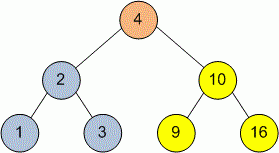Competitions

# Tree PreOrder Traversal

Implement a PreOrder traversal of a tree. In this traversal the root node is visited first, then the left subtree and finally the right subtree.

Definition of a tree:

// Java
class TreeNode
{
public:
int val;
TreeNode left;
TreeNode right;
TreeNode(int x) {
val = x;
left = NULL;
right = NULL;
};

// C++
class TreeNode
{
public:
int val;
TreeNode *left;
TreeNode *right;
TreeNode(int x) : val(x), left(NULL), right(NULL) {}
};


Implement function PreOrder that prints in one line the vertices of the tree in order of PreOrder traversal. Separate the vertices with one space.

// Java
void PreOrder(TreeNode tree)

// C++
void PreOrder(TreeNode *tree)


#### ExampleDuring the PreOrder traversal vertices will be printed in the next order: 4 2 1 3 10 9 16.

Time limit 1 second
Memory limit 128 MiB
Author Mykhailo Medvediev